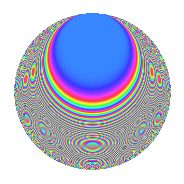# Properties

 Label 216.2.qLevel $216$ Weight $2$ Character orbit 216.q Rep. character $\chi_{216}(25,\cdot)$ Character field $\Q(\zeta_{9})$ Dimension $54$ Newform subspaces $2$ Sturm bound $72$ Trace bound $1$

# Related objects

## Defining parameters

 Level: $$N$$ $$=$$ $$216 = 2^{3} \cdot 3^{3}$$ Weight: $$k$$ $$=$$ $$2$$ Character orbit: $$[\chi]$$ $$=$$ 216.q (of order $$9$$ and degree $$6$$) Character conductor: $$\operatorname{cond}(\chi)$$ $$=$$ $$27$$ Character field: $$\Q(\zeta_{9})$$ Newform subspaces: $$2$$ Sturm bound: $$72$$ Trace bound: $$1$$ Distinguishing $$T_p$$: $$5$$

## Dimensions

The following table gives the dimensions of various subspaces of $$M_{2}(216, [\chi])$$.

Total New Old
Modular forms 240 54 186
Cusp forms 192 54 138
Eisenstein series 48 0 48

## Trace form

 $$54q + O(q^{10})$$ $$54q + 3q^{11} + 12q^{15} + 12q^{17} + 12q^{21} + 12q^{23} - 15q^{27} - 18q^{29} - 9q^{33} - 36q^{35} - 36q^{39} - 21q^{41} + 9q^{43} - 54q^{45} - 54q^{47} + 18q^{49} - 42q^{51} - 36q^{53} - 45q^{57} - 42q^{59} + 18q^{61} - 36q^{63} - 72q^{65} + 9q^{67} - 48q^{69} + 18q^{75} - 12q^{77} - 12q^{81} + 30q^{83} + 30q^{87} + 45q^{89} + 72q^{93} + 138q^{95} - 27q^{97} + 138q^{99} + O(q^{100})$$

## Decomposition of $$S_{2}^{\mathrm{new}}(216, [\chi])$$ into newform subspaces

Label Dim. $$A$$ Field CM Traces $q$-expansion
$$a_2$$ $$a_3$$ $$a_5$$ $$a_7$$
216.2.q.a $$24$$ $$1.725$$ None $$0$$ $$0$$ $$0$$ $$-3$$
216.2.q.b $$30$$ $$1.725$$ None $$0$$ $$0$$ $$0$$ $$3$$

## Decomposition of $$S_{2}^{\mathrm{old}}(216, [\chi])$$ into lower level spaces

$$S_{2}^{\mathrm{old}}(216, [\chi]) \cong$$ $$S_{2}^{\mathrm{new}}(27, [\chi])$$$$^{\oplus 4}$$$$\oplus$$$$S_{2}^{\mathrm{new}}(54, [\chi])$$$$^{\oplus 3}$$$$\oplus$$$$S_{2}^{\mathrm{new}}(108, [\chi])$$$$^{\oplus 2}$$

## Hecke characteristic polynomials

There are no characteristic polynomials of Hecke operators in the database